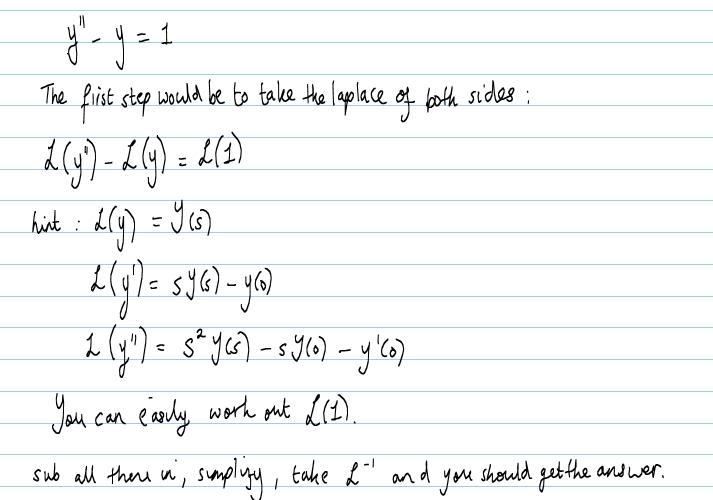# Laplace Transform Problem - Peacewise Functions

bathtub2007
Okay so this problem has been giving me nightmares ever since I laid eyes on it.

The problem: y'' - y = f(t), y(0) = 1, y'(0) = 0

f(t) = 1, 0 (less than equal to) t (less than) pi/2
f(t) = sin(t), t (greater than equal to) pi/2

Any help would be appreciated putting me in the right direction.

--Julie

xfunctionx
I think the best way forward would be:matticus
except f(t) isn't 1, read the post again. for piecewise functions use the unit step (heaviside) function.

Gold Member
You can rewrite f(t) in terms of the heaviside function as matticus said, then perform the transform.

$$f(t)=1+H(t-\frac{\pi}{2})+\left(sin(t)-1\right)H(t-\frac{\pi}{2})$$

Edit since latex isn't working...

f(t)=1+H(t-[pi/2])+[sin(t)-1]*H(t-[pi/2])

where H(t-[pi/2]) denotes the unit step function.

Maths.tuition
Hi I was thinking about the same approach as xfunctionx.

Thanks

Gold Member
maths.tuition welcome to PF.

If you are speaking of doing the same thing that xfunctionx did for bathtub's differential equation that would be wrong

f(t) = 1, 0 (less than equal to) t (less than) pi/2
f(t) = sin(t), t (greater than equal to) pi/2

does not simply equal 1 for all t, please note I already stated the proper form of the function(in terms of the unit step function) to get the the laplace transform for f(t)

"f(t)=1+H(t-[pi/2])+[sin(t)-1]*H(t-[pi/2])"

Last edited:
coomast
maths.tuition welcome to PF.

If you are speaking of doing the same thing that xfunctionx did for bathtub's differential equation that would be wrong

f(t) = 1, 0 (less than equal to) t (less than) pi/2
f(t) = sin(t), t (greater than equal to) pi/2

does not simply equal 1 for all t, please note I already stated the proper form of the function(in terms of the unit step function) to get the the laplace transform for f(t)

"f(t)=1+H(t-[pi/2])+[sin(t)-1]*H(t-[pi/2])"

I think this last expression is wrong. Musn't this be:

$$L\left{H(t)-H\left(t-\frac{\pi}{2}\right)+sin(t)H\left(t-\frac{\pi}{2}\right)\right}= L\left{H(t)-H\left(t-\frac{\pi}{2}\right)+cos\left(t-\frac{\pi}{2}\right)H\left(t-\frac{\pi}{2}\right)\right}$$

There seems to be something definitely wrong with the latex generation, it keeps posting things from older replies of other posts of me :grumpy:

coomast

Gold Member
You are right the correct function is

f(t)=1+H(t-[pi/2])+[sin(t)-2]*H(t-[pi/2])

one had to subtract 2 from sin and not 1 or else you would be left with 2-1+sin{t} = 1+sin(t)

thanks for catching that mistake

coomast
You are right the correct function is

f(t)=1+H(t-[pi/2])+[sin(t)-2]*H(t-[pi/2])

one had to subtract 2 from sin and not 1 or else you would be left with 2-1+sin{t} = 1+sin(t)

thanks for catching that mistake

OK, next attempt for a good latex formula. I think it is still not OK, I got:

$$L\left[H(t)-H\left(t-\frac{\pi}{2}\right)+sin(t)H\left(t-\frac{\pi}{2}\right)\right]= L\left[H(t)-H\left(t-\frac{\pi}{2}\right)+cos\left(t-\frac{\pi}{2}\right)H\left(t-\frac{\pi}{2}\right)\right]$$

coomast